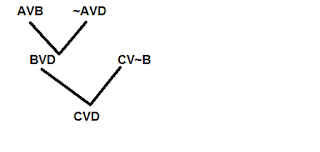1.1k views

The resolvent of the set of clauses $(A \vee B, \sim A \vee D, C \vee \sim B)$ is

1. $A \vee​ B$
2. $C \vee​ D$
3. $A \vee​ C$
4. $A \vee​ D$

recategorized | 1.1k views

ans will be B) C   D

A & ~A will cancel out  and so will B and ~B

hence ans

by Boss (49.4k points)
selected
0

Plz Tell the procedure of finding resolvent.

0
+2

Robinson in 1965 introduced the resolution principle, which can be directly
applied to any set of clauses. The principal is
"Given any two clauses A and B, if there is a literal P1 in A which has a
complementary literal P2 in B, delete P1 & P2 from A and B and construct a
disjunction of the remaining clauses. The clause so constructed is called
resolvent of A and B."
For example, consider the following clauses
A: P V Q V R
B: p' V Q V R
C: Q' V R
Clause A has the literal P which is complementary to `P in B. Hence both of
them deleted and a resolvent (disjunction of A and B after the complementary
clauses are removed) is generated. That resolvent has again a literal Q whose
negation is available in C. Hence resolving those two, one has the final
resolvent.
A: P V Q V R (given in the problem)
B: p' V Q V R (given in the problem)
D: Q V R (resolvent of A and B)
C: Q' V R (given in the problem)
E: R (resolvent of C and D)

Resolvent is the outcome of applying the resolution rule.

$\frac{{A\lor B , \thicksim A \lor B, C \lor \thicksim D}}{C \lor D}$

Hence,Option(B) $C \lor D$.

by Boss (41.3k points)
+1 voteans is B

by Active (1.9k points)